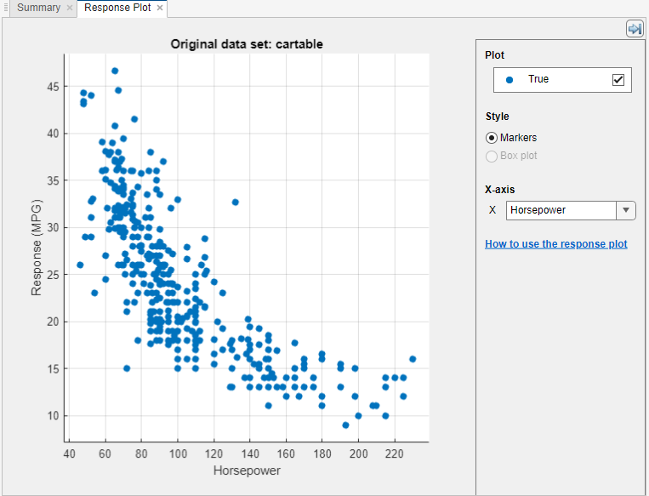Feature Selection and Feature Transformation Using Regression Learner App

Investigate Features in the Response Plot

In Regression Learner, use the response plot to try to identify predictors that are useful for predicting the response. To visualize the relation between different predictors and the response, under X-axis, select different variables in the X list.

Before you train a regression model, the response plot shows the training data. If you have trained a regression model, then the response plot also shows the model predictions.

Observe which variables are associated most clearly with the response. When you plot the carbig data set, the predictor Horsepower shows a clear negative association with the response.

Look for features that do not seem to have any association with the response and useto remove those features from the set of used predictors.You can export the response plots you create in the app to figures. See Export Plots in Regression Learner App.

Select Features to Include

In Regression Learner, you can specify different features (or predictors) to include in the model. See if you can improve models by removing features with low predictive power. If data collection is expensive or difficult, you might prefer a model that performs satisfactorily with fewer predictors.

1. On the Regression Learner tab, in the Features section, click.

2. In the Feature Selection dialog box, clear the check boxes for the predictors you want to exclude, and then click .

3. Click to train a new model using the new predictor options.

4. Observe the new model in the Models pane. The Current Model Summary pane displays how many predictors are excluded.

5. Check which predictors are included in a trained model. Click the model in the Models pane and look at the check boxes in the Feature Selection window.

6. Try to improve the model by including different features.

For an example using feature selection, see Train Regression Trees Using Regression Learner App.

Transform Features with PCA in Regression Learner

Use principal component analysis (PCA) to reduce the dimensionality of the predictor space. Reducing the dimensionality can create regression models in Regression Learner that help prevent overfitting. PCA linearly transforms predictors to remove redundant dimensions, and generates a new set of variables called principal components.

1. On the Regression Learner tab, in the Features section, select .

2. In the Advanced PCA Options dialog box, select the Enable PCA check box, and then click .

3. Click again. The pca function transforms your selected features before training the model.

By default, PCA keeps only the components that explain 95% of the variance. In the Advanced PCA Options dialog box, you can change the percentage of variance to explain by selecting the Explained variance value. A higher value risks overfitting, while a lower value risks removing useful dimensions.

4. Manually limit the number of PCA components. In the Component reduction criterion list, select Specify number of components. Select the Number of numeric components value. The number of components cannot be larger than the number of numeric predictors. PCA is not applied to categorical predictors.

You can check PCA Options for trained models in the Current Model Summary pane. For example:

PCA is keeping enough components to explain 95% variance.
After training, 2 components were kept.
Explained variance per component (in order): 92.5%, 5.3%, 1.7%, 0.5%
Check the explained variance percentages to decide whether to change the number of components.